# ML Aggarwal Class 7 Solutions for ICSE Maths Chapter 4 Exponents and Powers Ex 4.2

## ML Aggarwal Class 7 Solutions for ICSE Maths Chapter 4 Exponents and Powers Ex 4.2

Question 1.
Using laws of exponents, simplify and write the following in the exponential form:Solution:Question 2.
Simplify and write the following in the exponential form:
(i) 53 × 57 × 512
(ii) a5 × a3 × a7
(iii) (712 × 73) ÷ 74
Solution:Question 3.
Simplify and write the following in the exponential form:
(i) (22)100
(ii) ((-7)6)5
(iii) (32)5 × (34)7
Solution: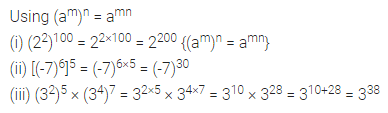Question 4.
Simplify and write in the exponential form: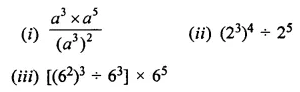Solution: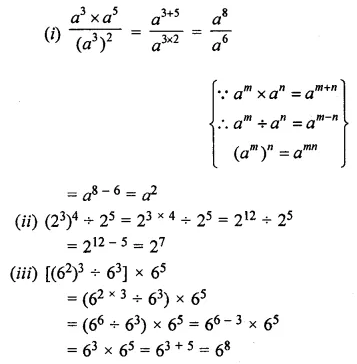Question 5.
Simplify and write in the exponential form: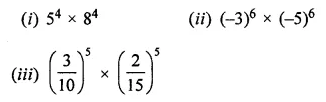Solution:Question 6.
Simplify and express each of the following in the exponential form: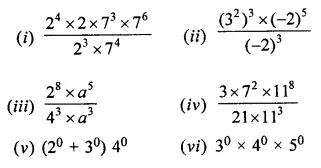Solution: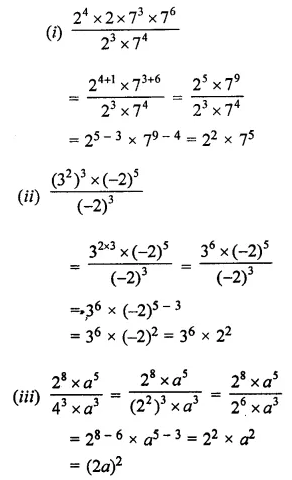Question 7.
Express each of the following rational numbers in the exponential form:Solution:Question 8.
Simplify the following: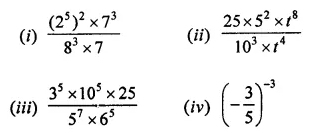Solution: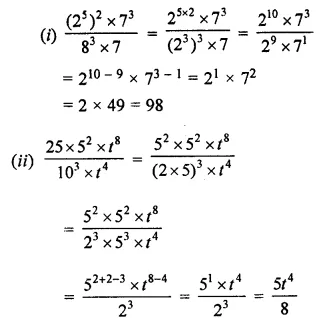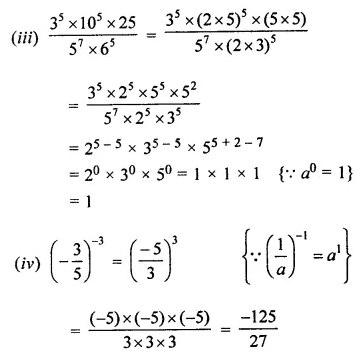Question 9.
Simplify the following:Solution: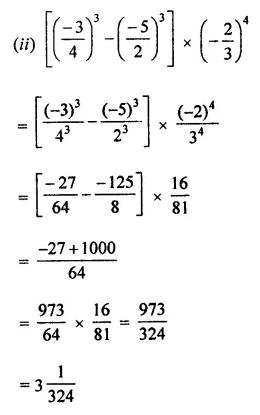Question 10.
Simplify the following: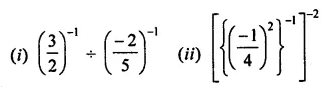Solution: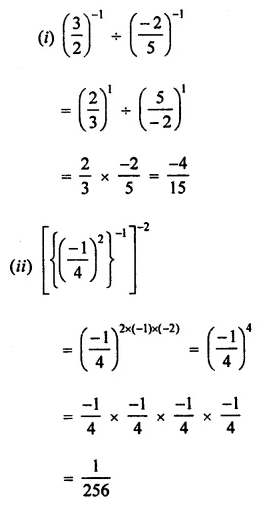Question 11.
Simplify: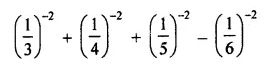Solution:Question 12.
Express each of the following as a product of prime factors in the exponential form:
(i) 108 × 192
(ii) 729 × 64
(iii) 384 × 147
Solution: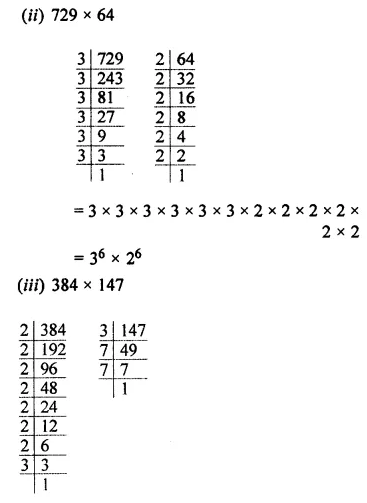Question 13.
Simplify and write the following in the exponential form:
(i) 33 × 22 + 22 × 50
(ii) 92 + 112 – 22 × 3 × 170
Solution: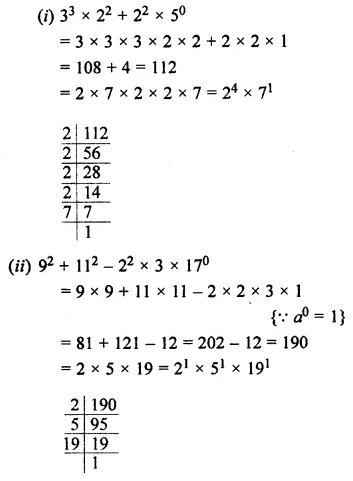Question 14.
(i) By what number should we multiply 34 so that the product is 37?
(ii) By what number should be multiple (-6)-1 so that the product is 10-1?
Solution: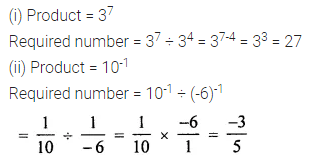Question 15.Solution: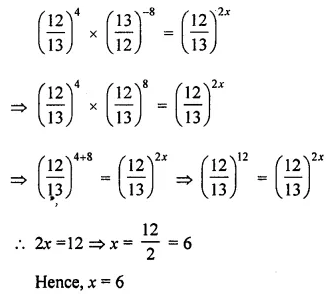### 2 thoughts on “ML Aggarwal Class 7 Solutions for ICSE Maths Chapter 4 Exponents and Powers Ex 4.2”

1.It’s soooooo good

•Fantastic